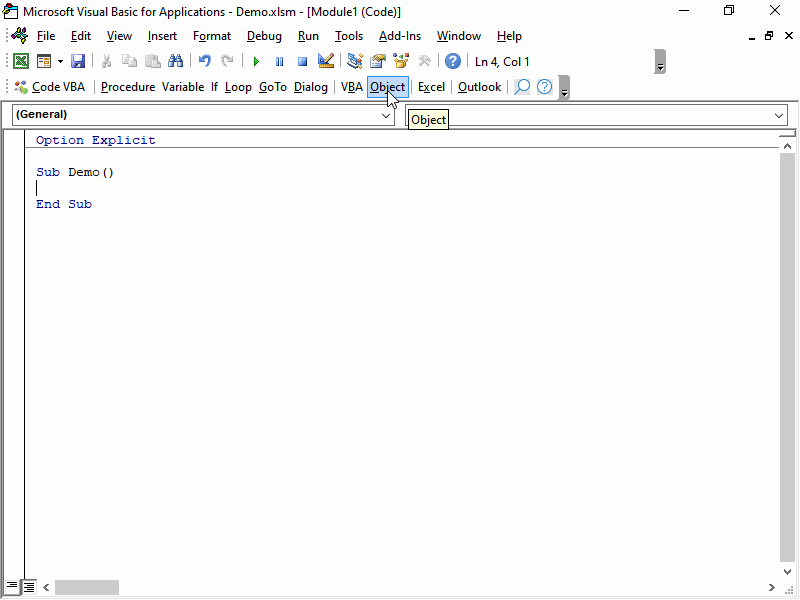﻿ Hide a column using VBA in Excel

# Hide columns using VBA in Excel

## Hiding one or more columns on a worksheet

Hiding a column can be simple. Below code hides column C on the active worksheet. If you set the property `Hidden` to `False` the column becomes visible again.

``Columns("D").Hidden = True``

Below code hides multiple columns (D. E and F).

``Columns("D:F").Hidden = True``

## Hiding columns in a rangeWhen starting with a range which is not a complete column you will get run-time error '1004': Unable to set the Hidden property of the Range class.

``````Dim rng As Range: Set rng = Application.Range("B2")
rng.Hidden = True``````

You can fix this by applying EntireColumn to the range:

``````Dim rng As Range: Set rng = Application.Range("B2")
rng.EntireColumn.Hidden = True``````

## Make a column xlVeryHidden

xlVeryHidden is not supported for Columns. A workaround is to hide it and then protect the sheet.

## Getting the code using Code VBA

The animation below shows how the Code VBA add-in helps with coding this task.

1. Select Object 'Sheet1' - here we want to hide columns in the same workbook as the one that has the code module
2. Select Columns - the collection you want to work on
3. Select Properties and Hidden - the visibility is determined using the 'Hidden' property
4. Let the property have the value 'True'
5. Specify the subset of the Columns you want to hide